Electron. J. Differential Equations, Vol. 2018 (2018), No. 81, pp. 1-11.

### Liouville-type theorems for stable solutions of singular quasilinear elliptic equations in R^N Caisheng Chen, Hongxue Song, Hongwei Yang

Abstract:
We prove a Liouville-type theorem for stable solution of the singular quasilinear elliptic equations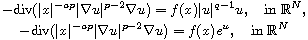where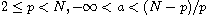and the function f(x) is continuous and nonnegative in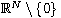such that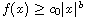as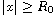, with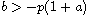and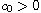. The results hold for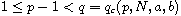in the first equation, and for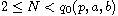in the second equation. Here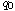and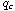are exponents, which are always larger than the classical critical ones and depend on the parameters a,b.

Submitted June 26, 2017. Published March 22, 2018.
Math Subject Classifications: 35J60, 35B53, 35B33, 35B45.
Key Words: Singular quasilinear elliptic equation; stable solutions; critical exponents; Liouville type theorems.

Show me the PDF file (261 KB), TEX file for this article.Caisheng Chen College of Science, Hohai University Nanjing 210098, China email: cshengchen@hhu.edu.cn Hongxue Song College of Science, Hohai University Nanjing 210098, China email: songhx@njupt.edu.cn Hongwei Yang College of Mathematics and System Science Shandong University of Science and Technology Qingdao 266590, China email: hwyang1979@163.com